• python数据动态可视化
万次阅读
2018-06-25 20:10:20

# python数据动态可视化

高频实时数据可视化，做一个简单记录：

• 参考资料
• 代码块实例

## 代码示例

参考资料例子为主题，根据需求进行修改。

### 实例1

代码块语法遵循标准markdown代码，例如：

import numpy as np
import time
import matplotlib
matplotlib.use('GTKAgg')
from matplotlib import pyplot as plt
import random

def randomwalk(dims=(256, 256), n=20, sigma=5, alpha=0.95, seed=1):
""" A simple random walk with memory """

r, c = dims
gen = np.random.RandomState(seed)
#pos = gen.rand(2, n) * ((r,), (c,))
old_delta = gen.randn(2, n) * sigma
pos=[[]]
while True:
#delta = (1. - alpha) * gen.randn(2, n) * sigma + alpha * old_delta
#pos += delta
#for ii in xrange(n):
#    if not (0. <= pos[0, ii] < r):
#        pos[0, ii] = abs(pos[0, ii] % r)
#    if not (0. <= pos[1, ii] < c):
#        pos[1, ii] = abs(pos[1, ii] % c)
#old_delta = delta
a=random.randint(1,1000)
b=random.randint(1,1000)

pos.append(a)
yield (a ,b)#pos
#yield pos

def run(niter=10000, doblit=True):
"""
Display the simulation using matplotlib, optionally using blit for speed
"""

fig, ax = plt.subplots(1, 1)
ax.set_aspect('equal')
ax.set_xlim(0, 255)
ax.set_ylim(0, 255)
ax.hold(True)
#rw = randomwalk()
#x, y = randomwalk()#rw.next()
a=random.randint(1,1000)
b=random.randint(1,1000)
x=[]
y=[]
x.append(a)#random.randint(1,1000)
y.append(b)#random.randint(1,1000)

plt.show(False)
plt.draw()

if doblit:
# cache the background
background = fig.canvas.copy_from_bbox(ax.bbox)

points = ax.plot(x, y, 'o')
fig.canvas.draw()

tic = time.time()

for ii in xrange(niter):

# update the xy data
#x, y = rw.next()
a=random.randint(1,1000)
b=random.randint(1,1000)
x.append(a)
y.append(b)
points.set_data(x, y)

if doblit:
# restore background
fig.canvas.restore_region(background)

# redraw just the points

ax.draw_artist(points)

# fill in the axes rectangle
fig.canvas.blit(ax.bbox)

else:
# redraw everything
fig.canvas.draw()

plt.close(fig)
print "Blit = %s, average FPS: %.2f" % (
str(doblit), niter / (time.time() - tic))

if __name__ == '__main__':
run(doblit=False)
run(doblit=True)

### 说明

保留背景绘制数据，相比绘制整幅图提高10倍左右，随着数据增加提高效率降低。

更多相关内容
• Python为基础进行数据分析，Matplotlib存在不够美观、静态性、不易分享等缺点，限制了Python在数据可视化中的发展。为了解决这个问题，新型的动态可视化开源模块Plotly应运而生。由于Plotly具有动态、美观、易用、...编程语言
• Python 和 Echart 实现的酷炫可视化大屏。 详细手册参考我的博文： https://blog.csdn.net/lildkdkdkjf/article/details/107083274
• 主要为大家详细介绍了python可视化动态CPU性能监控，具有一定的参考价值，感兴趣的小伙伴们可以参考一下
• Python数据可视化编程实战是一本使用Python实现数据...《Python数据可视化编程实战》适合那些对Python编程有一定基础的开发人员，可以帮助读者从头开始了解数据、数据格式、数据可视化，并学会使用Python可视化数据。
• json数据转 csv import json import time import csv file = open('DXYArea-TimeSeries.json','r',encoding='utf-8') infos = json.load(file) with open('data.csv','a',newline='') as f: writer = csv....
• 【印度】科斯·拉曼著 完整版 《Python数据可视化》介绍了利用Python实现数据可视化。并介绍了数据、信息与知识之间的关系。书中涉及的可视化过程应用了大量流行的Python库，你会学到采用Numpy、Scipy、IPython、...大数据
• 基于Python可视化数据分析系统采Django框架进行开发，功能包括缺失值处理、异常值处理、重复值处理、探索性数据分析、决策树、线性回归、KNN分类、k-means聚类、PCA数据降维。数据可视化功能使用Echart进行设计，...
• 基于Python可视化数据分析平台设计与实现.pdf
• 基于Python可视化数据分析平台设计与实现 (1).pdf
• 可以通过设置页面动画，来制作动态可视化图表。 官网地址：Tableau。 二、Echarts 百度旗下基于 JavaScript 的开源 Web 可视化工具，Apache 顶级项目。Echarts 目前有 Python 接口-Pyecharts，可以通过 pip 安装...

## 一、Tableau

• 世界知名的 BI 工具，以超强的可视化能力著称。它已经成为商业 BI 界的 TOP 选手，很多大型公司像阿里、谷歌都在使用，能快速搭建数据系统。
• 可以通过设置页面动画，来制作动态可视化图表。
• 官网地址：Tableau## 二、Echarts

• 百度旗下基于 JavaScript 的开源 Web 可视化工具，Apache 顶级项目。Echarts 目前有 Python 接口-Pyecharts，可以通过 pip 安装。
• 在网页上看到的各种炫酷的动态图表，Echarts 都能搞定。
• 官网地址：Echarts## 三、Flourish

• 一个专门制作动态数据的可视化网站，提供了多少种数据展示场景。
• 不用下载软件，直接在浏览器上就可以通过 Flourish 制作图表，非常方便。
• 在抖音上常看到的动态排名视频，就可以用 Flourish 来制作。
• 官方地址：Flourish## 四、Python

• Python 不用多说了，全能选手，是数据科学领域的专业编程语言，可视化也是它的强项。它拥有 matplotlib、plotly、bokeh、pyecharts 等多种动态可视化库，可以高定制化完成各种展示。
• 官网地址：Python## 五、kepler.gl

• 地图可视化爱好者的福音，这个工具很少被人知道，但非常好用。它是 Uber 开发的地图可视化工具，能对大规模地理数据进行动态渲染，而且地图场景非常丰富。
• 官网地址：kepler.gl展开全文Tableau Echarts Flourish
• 《Python数据可视化编程实战》是一本使用Python实现...《Python数据可视化编程实战》适合那些对Python编程有一定基础的开发人员，可以帮助读者从头开始了解数据、数据格式、数据可视化，并学会使用Python可视化数据。
• python爬虫，并将数据进行可视化分析，数据可视化包含饼图、柱状图、漏斗图、词云、另附源代码和报告书。
• 利用 Python3.5+PyCharm+PyQt 编写可视化界面。简单示例
• Python数据可视化编程实战 一书的示例代码，书名英文为python Data visualization cookbook
• 你可能经常会在公司前台、展会、路演时看到可视化数据的交互大屏。今天我们就来用 python 做一个动态可视化的交互大屏图。一、项目背景随着科技的飞速发展，数据呈现爆发式的增长，任何人都摆...你可能经常会在公司前台、展会、路演时看到可视化数据的交互大屏。今天我们就来用 python 做一个动态可视化的交互大屏图。

一、项目背景

随着科技的飞速发展，数据呈现爆发式的增长，任何人都摆脱不了与数据打交道，社会对于“数据”方面的人才需求也在不断增大。因此了解当下企业究竟需要招聘什么样的人才？需要什么样的技能？不管是对于在校生，还是对于求职者来说，都显得很有必要。

本文基于这个问题，针对51job招聘网站，爬取了全国范围内大数据、数据分析、数据挖掘、机器学习、人工智能等相关岗位的招聘信息。

分析比较了不同岗位的薪资、学历要求；分析比较了不同区域、行业对相关人才的需求情况；分析比较了不同岗位的知识、技能要求等。

二、效果展示

本次数据分析的特点在于：使用Tableau将数据分析的结果做成 可视化交互大屏，效果如下：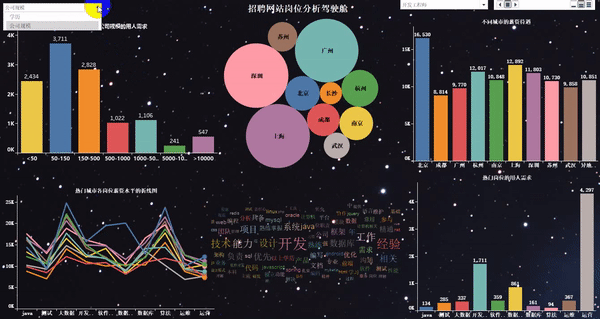三、爬取数据

基于51job招聘网站，我们搜索全国对于“数据”岗位的需求，大概有2000页。

1. 爬取岗位：大数据、数据分析、机器学习、人工智能等相关岗位。

2. 爬取字段：公司名、岗位名、工作地址、薪资、发布时间、工作描述、公司类型、员工人数、所属行业。

我们爬取的字段，既有一级页面的相关信息，还有二级页面的部分信息，大概爬取思路为：先针对某一页数据的一级页面做一个解析，然后再进行二级页面做一个解析，最后再进行翻页操作

使用工具：Python+requests+lxml+pandas+time+Xpath

1.导入相关库

import requests
import pandas as pd
from pprint import pprint
from lxml import etree
import time
import warnings
warnings.filterwarnings("ignore")


2.关于翻页的说明

# 第一页的特点
https://search.51job.com/list/000000,000000,0000,00,9,99,%25E6%2595%25B0%25E6%258D%25AE,2,1.html?
# 第二页的特点
https://search.51job.com/list/000000,000000,0000,00,9,99,%25E6%2595%25B0%25E6%258D%25AE,2,2.html?
# 第三页的特点
https://search.51job.com/list/000000,000000,0000,00,9,99,%25E6%2595%25B0%25E6%258D%25AE,2,3.html?


注意：通过对于页面的观察，可以看出，就一个地方的数字变化了，因此只需要做字符串拼接，然后循环爬取即可。

3.完整的爬取代码

import requests
import pandas as pd
from pprint import pprint
from lxml import etree
import time
import warnings
warnings.filterwarnings("ignore")

for i in range(1,1501):
print("正在爬取第" + str(i) + "页的数据")
url_pre = "https://search.51job.com/list/000000,000000,0000,00,9,99,%25E6%2595%25B0%25E6%258D%25AE,2,"
url_end = ".html?"
url = url_pre + str(i) + url_end
'User-Agent': 'Mozilla/5.0 (Windows NT 10.0; Win64; x64) AppleWebKit/537.36 (KHTML, like Gecko) Chrome/73.0.3683.86 Safari/537.36'
}
web.encoding = "gbk"
dom = etree.HTML(web.text)
# 1、岗位名称
job_name = dom.xpath('//div[@class="dw_table"]/div[@class="el"]//p/span/a[@target="_blank"]/@title')
# 2、公司名称
company_name = dom.xpath('//div[@class="dw_table"]/div[@class="el"]/span[@class="t2"]/a[@target="_blank"]/@title')
# 3、工作地点
# 4、工资
salary_mid = dom.xpath('//div[@class="dw_table"]/div[@class="el"]/span[@class="t4"]')
salary = [i.text for i in salary_mid]
# 5、发布日期
release_time = dom.xpath('//div[@class="dw_table"]/div[@class="el"]/span[@class="t5"]/text()')
# 6、获取二级网址url
deep_url = dom.xpath('//div[@class="dw_table"]/div[@class="el"]//p/span/a[@target="_blank"]/@href')
RandomAll = []
JobDescribe = []
CompanyType = []
CompanySize = []
Industry = []
for i in range(len(deep_url)):
web_test.encoding = "gbk"
dom_test = etree.HTML(web_test.text)
# 7、爬取经验、学历信息，先合在一个字段里面，以后再做数据清洗。命名为random_all
# 8、岗位描述性息
job_describe = dom_test.xpath('//div[@class="tBorderTop_box"]//div[@class="bmsg job_msg inbox"]/p/text()')
# 9、公司类型
company_type = dom_test.xpath('//div[@class="tCompany_sidebar"]//div[@class="com_tag"]/p/@title')
# 10、公司规模(人数)
company_size = dom_test.xpath('//div[@class="tCompany_sidebar"]//div[@class="com_tag"]/p/@title')
# 11、所属行业(公司)
industry = dom_test.xpath('//div[@class="tCompany_sidebar"]//div[@class="com_tag"]/p/@title')
# 将上述信息保存到各自的列表中
RandomAll.append(random_all)
JobDescribe.append(job_describe)
CompanyType.append(company_type)
CompanySize.append(company_size)
Industry.append(industry)
# 为了反爬，设置睡眠时间
time.sleep(1)
# 由于我们需要爬取很多页，为了防止最后一次性保存所有数据出现的错误，因此，我们每获取一夜的数据，就进行一次数据存取。
df = pd.DataFrame()
df["岗位名称"] = job_name
df["公司名称"] = company_name
df["工资"] = salary
df["发布日期"] = release_time
df["经验、学历"] = RandomAll
df["公司类型"] = CompanyType
df["公司规模"] = CompanySize
df["所属行业"] = Industry
df["岗位描述"] = JobDescribe
# 这里在写出过程中，有可能会写入失败，为了解决这个问题，我们使用异常处理。
try:
except:
print("当页数据写入失败")
time.sleep(1)
print("数据爬取完毕，是不是很开心！！！")


这里可以看到，我们爬取了1000多页的数据做最终的分析。因此每爬取一页的数据，做一次数据存储，避免最终一次性存储导致失败。同时根据自己的测试，有一些页数进行数据存储，会导致失败，为了不影响后面代码的执行，我们使用了“try-except”异常处理。

在一级页面中，我们爬取了“岗位名称”，“公司名称”，“工作地点”，“工资”，“发布日期”，“二级网址的url”这几个字段。

在二级页面中，我们爬取了“经验、学历信息”，“岗位描述”，“公司类型”，“公司规模”，“所属行业”这几个字段。

四、数据预处理

从爬取到的数据中截取部分做了一个展示，可以看出数据很乱。

杂乱的数据并不利于我们的分析，因此需要根据研究的目标做一个数据预处理，得到我们最终可以用来做可视化展示的数据。

1.相关库的导入及数据的读取

df = pd.read_csv(r"G:\8泰迪\python_project\51_job\job_info1.csv",engine="python",header=None)
# 为数据框指定行索引
df.index = range(len(df))
# 为数据框指定列索引
df.columns = ["岗位名","公司名","工作地点","工资","发布日期","经验与学历","公司类型","公司规模","行业","工作描述"]


2.数据去重

我们认为一个公司的公司名和和发布的岗位名一致，就看作是重复值。因此，使用drop_duplicates(subset=[])函数，基于“岗位名”和“公司名”做一个重复值的剔除。

# 去重之前的记录数
print("去重之前的记录数",df.shape)
# 记录去重
df.drop_duplicates(subset=["公司名","岗位名"],inplace=True)
# 去重之后的记录数
print("去重之后的记录数",df.shape)


3.岗位名字段的处理

1）岗位名字段的探索

df["岗位名"].value_counts()
df["岗位名"] = df["岗位名"].apply(lambda x:x.lower())


首先我们对每个岗位出现的频次做一个统计，可以看出“岗位名字段”太杂乱，不便于我们做统计分析。接着我们将岗位名中的大写英文字母统一转换为小写字母，也就是说“AI”和“Ai”属于同一个东西。

2）构造想要分析的目标岗位，做一个数据筛选

job_info.shape
target_job = ['算法', '开发', '分析', '工程师', '数据', '运营', '运维']
index = [df["岗位名"].str.count(i) for i in target_job]
index = np.array(index).sum(axis=0) > 0
job_info = df[index]
job_info.shape


首先我们构造了如上七个目标岗位的关键字眼。然后利用count()函数统计每一条记录中，是否包含这七个关键字眼，如果包含就保留这个字段;不过不包含就删除这个字段，最后查看筛选之后还剩余多少条记录。

3）目标岗位标准化处理

由于目标岗位太杂乱，我们需要统一一下

job_list = ['数据分析', "数据统计","数据专员",'数据挖掘', '算法',
'大数据','开发工程师', '运营', '软件工程', '前端开发',
'深度学习', 'ai', '数据库', '数据库', '数据产品',
'客服', 'java', '.net', 'andrio', '人工智能', 'c++',
'数据管理',"测试","运维"]
job_list = np.array(job_list)
def rename(x=None,job_list=job_list):
index = [i in x for i in job_list]
if sum(index) > 0:
return job_list[index]
else:
return x
job_info["岗位名"] = job_info["岗位名"].apply(rename)
job_info["岗位名"].value_counts()
# 数据统计、数据专员、数据分析统一归为数据分析
job_info["岗位名"] = job_info["岗位名"].apply(lambda x:re.sub("数据专员","数据分析",x))
job_info["岗位名"] = job_info["岗位名"].apply(lambda x:re.sub("数据统计","数据分析",x))


首先我们定义了一个想要替换的目标岗位job_list，将其转换为ndarray数组。然后定义一个函数，如果某条记录包含job_list数组中的某个关键词，那么就将该条记录替换为这个关键词，如果某条记录包含job_list数组中的多个关键词，我们只取第一个关键词替换该条记录。接着使用value_counts()函数统计一下替换后的各岗位的频次。最后，我们将“数据专员”、“数据统计”统一归为“数据分析”。

4.工资水平字段的处理

工资水平字段的数据类似于“20-30万/年”、“2.5-3万/月”和“3.5-4.5千/月”这样的格式。我们需要做一个统一的变化，将数据格式转换为“元/月”，然后取出这两个数字，求一个平均值。

job_info["工资"].str[-1].value_counts()
job_info["工资"].str[-3].value_counts()

index1 = job_info["工资"].str[-1].isin(["年","月"])
index2 = job_info["工资"].str[-3].isin(["万","千"])
job_info = job_info[index1 & index2]

def get_money_max_min(x):
try:
if x[-3] == "万":
z = [float(i)*10000 for i in re.findall("[0-9]+\.?[0-9]*",x)]
elif x[-3] == "千":
z = [float(i) * 1000 for i in re.findall("[0-9]+\.?[0-9]*", x)]
if x[-1] == "年":
z = [i/12 for i in z]
return z
except:
return x

salary = job_info["工资"].apply(get_money_max_min)
job_info["最低工资"] = salary.str
job_info["最高工资"] = salary.str
job_info["工资水平"] = job_info[["最低工资","最高工资"]].mean(axis=1)


首先我们做了一个数据筛选，针对于每一条记录，如果最后一个字在“年”和“月”中，同时第三个字在“万”和“千”中，那么就保留这条记录，否则就删除。接着定义了一个函数，将格式统一转换为“元/月”。最后将最低工资和最高工资求平均值，得到最终的“工资水平”字段。

5.工作地点字段的处理

由于整个数据是关于全国的数据，涉及到的城市也是特别多。我们需要自定义一个常用的目标工作地点字段，对数据做一个统一处理。

#job_info["工作地点"].value_counts()
address_list = ['北京', '上海', '广州', '深圳', '杭州', '苏州', '长沙',
'武汉', '天津', '成都', '西安', '东莞', '合肥', '佛山',
'宁波', '南京', '重庆', '长春', '郑州', '常州', '福州',
'沈阳', '济南', '宁波', '厦门', '贵州', '珠海', '青岛',
'中山', '大连','昆山',"惠州","哈尔滨","昆明","南昌","无锡"]

index = [i in x for i in address_list]
if sum(index) > 0:
else:
return x
job_info["工作地点"] = job_info["工作地点"].apply(rename)


首先我们定义了一个目标工作地点列表，将其转换为ndarray数组。接着定义了一个函数，将原始工作地点记录，替换为目标工作地点中的城市。

6.公司类型字段的处理

这个很容易，就不详细说明了。

job_info.loc[job_info["公司类型"].apply(lambda x:len(x)<6),"公司类型"] = np.nan
job_info["公司类型"] = job_info["公司类型"].str[2:-2]


7.行业字段的处理

每个公司的行业字段可能会有多个行业标签，但是我们默认以第一个作为该公司的行业标签。

# job_info["行业"].value_counts()
job_info["行业"] = job_info["行业"].apply(lambda x:re.sub(",","/",x))
job_info.loc[job_info["行业"].apply(lambda x:len(x)<6),"行业"] = np.nan
job_info["行业"] = job_info["行业"].str[2:-2].str.split("/").str


8.经验与学历字段的处理

关于这个字段的数据处理，我很是思考了一会儿，不太好叙述，放上代码自己下去体会。

job_info["学历"] = job_info["经验与学历"].apply(lambda x:re.findall("本科|大专|应届生|在校生|硕士",x))
def func(x):
if len(x) == 0:
return np.nan
elif len(x) == 1 or len(x) == 2:
return x
else:
return x
job_info["学历"] = job_info["学历"].apply(func)


9.工作描述字段的处理

对于每一行记录，我们去除停用词以后，做一个jieba分词。

with open(r"G:\8泰迪\python_project\51_job\stopword.txt","r") as f:
stopword = stopword.split()
stopword = stopword + ["任职","职位"," "]

job_info["工作描述"] = job_info["工作描述"].str[2:-2].apply(lambda x:x.lower()).apply(lambda x:"".join(x))\
.apply(jieba.lcut).apply(lambda x:[i for i in x if i not in stopword])
job_info.loc[job_info["工作描述"].apply(lambda x:len(x) < 6),"工作描述"] = np.nan


10.公司规模字段的处理

#job_info["公司规模"].value_counts()
def func(x):
if x == "['少于50人']":
return "<50"
elif x == "['50-150人']":
return "50-150"
elif x == "['150-500人']":
return '150-500'
elif x == "['500-1000人']":
return '500-1000'
elif x == "['1000-5000人']":
return '1000-5000'
elif x == "['5000-10000人']":
return '5000-10000'
elif x == "['10000人以上']":
return ">10000"
else:
return np.nan
job_info["公司规模"] = job_info["公司规模"].apply(func)


11.构造新数据

我们针对最终清洗干净的数据，选取需要分析的字段，做一个数据存储。

feature = ["公司名","岗位名","工作地点","工资水平","发布日期","学历","公司类型","公司规模","行业","工作描述"]
final_df = job_info[feature]
final_df.to_excel(r"G:\8泰迪\python_project\51_job\词云图.xlsx",encoding="gbk",index=None)


五、字段的特殊处理

由于我们之后需要针对不同的岗位名做不同的词云图处理，并且是在tableau中做可视化展示，因此我们需要按照岗位名分类，求出不同岗位下各关键词的词频统计。

import numpy as np
import pandas as pd
import re
import jieba
import warnings
warnings.filterwarnings("ignore")

df

def get_word_cloud(data=None, job_name=None):
words = []
describe = data['工作描述'][data['岗位名'] == job_name].str[1:-1]
describe.dropna(inplace=True)
[words.extend(i.split(',')) for i in describe]
words = pd.Series(words)
word_fre = words.value_counts()
return word_fre

zz = ['数据分析', '算法', '大数据','开发工程师', '运营', '软件工程','运维', '数据库','java',"测试"]
for i in zz:
word_fre = get_word_cloud(data=df, job_name='{}'.format(i))
word_fre = word_fre[1:].reset_index()[:100]
word_fre["岗位名"] = pd.Series("{}".format(i),index=range(len(word_fre)))


六、tableau可视化展示

1. 热门城市的用人需求TOP10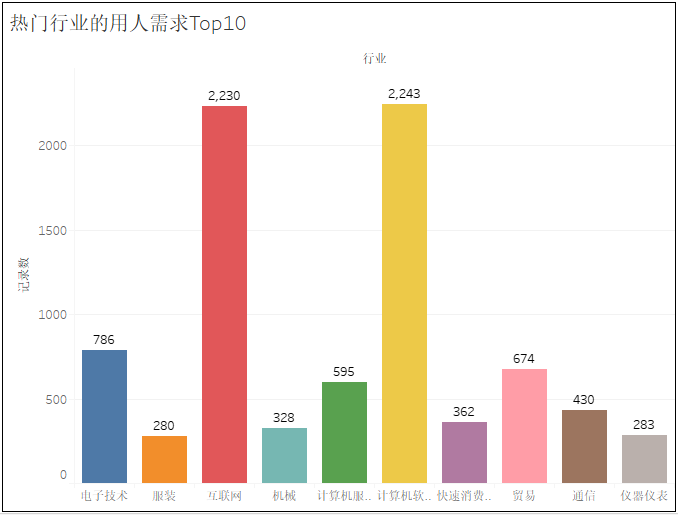2. 热门城市的岗位数量TOP10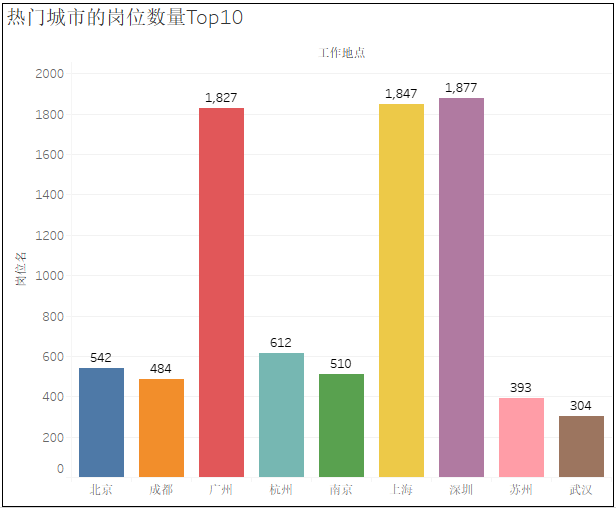3. 不同工作地点岗位数量的气泡图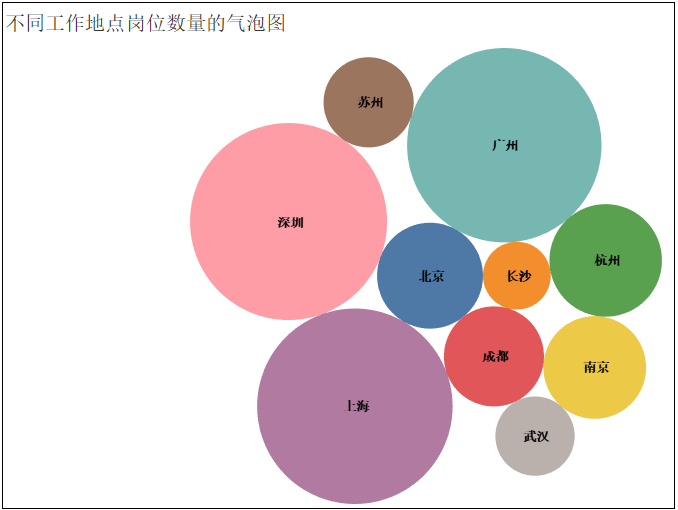4. 热门岗位的薪资待遇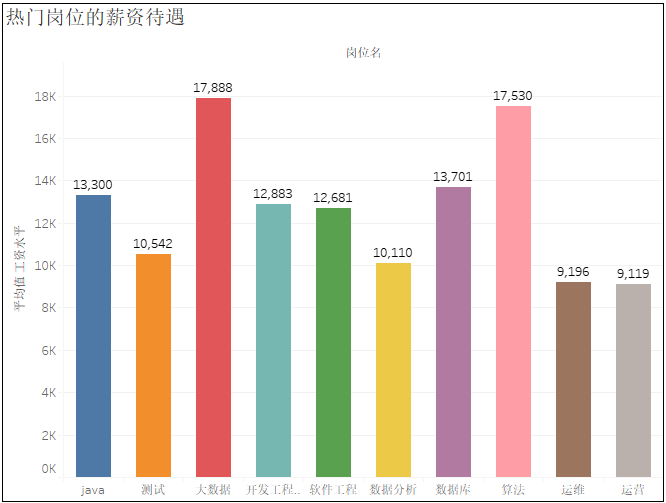5. 热门行业的薪资待遇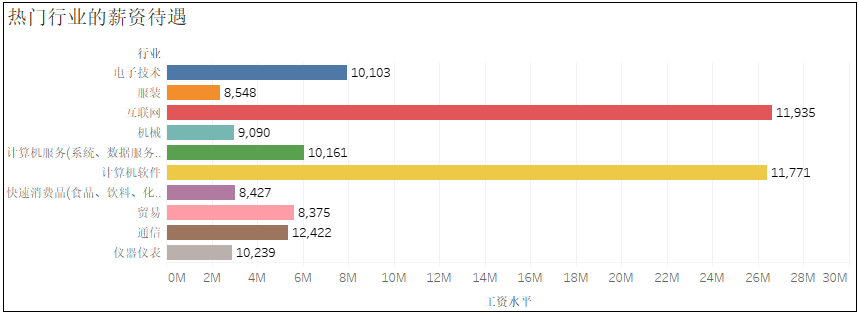6. 可视化大屏的“动态”展示这里最终就不做结论分析了，因为结论通过上图，就可以很清晰的看出来。想学习实践的同学可以下载源码自己分析哦！

源码链接：

https://pan.baidu.com/s/1ZF_r8FKGeYxalqvz25IQwA

提取码：hbix

作者：黄伟呢

来源：凹凸数据

不同的是，print和pprint是python的内置模块，而 beeprint 需要额外安装。

延伸阅读

Python：

技能GET：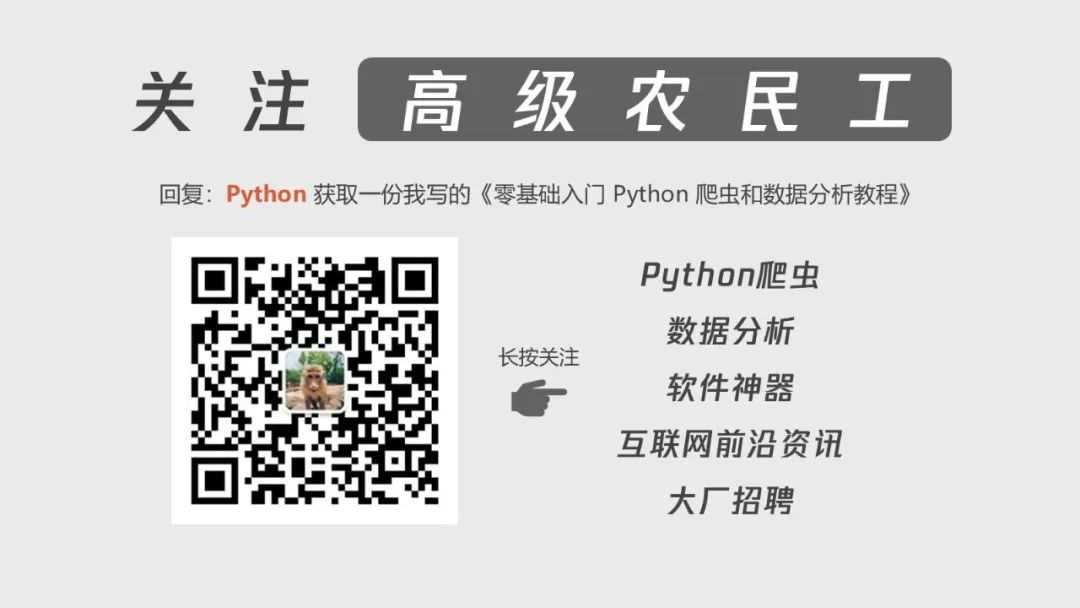展开全文• Python 和 Echart 实现的酷炫可视化大屏。 详细手册参考我的博文： https://blog.csdn.net/lildkdkdkjf/article/details/119801899
• 程序利用python numpy和matplotlib库实现了简单的梯度下降算法并对其进行可视化。程序通过简单的可视化过程解释了梯度下降的原理，供大家学习参考。注：并不是反向传播的实现，而是对梯度下降原理的解释，请根据需要...
• Python编写的学生成绩管理系统具有可视化界面
• 《Python数据可视化编程实战》适合那些对Python编程有一定基础的开发人员，可以帮助读者从头开始了解数据、数据格式、数据可视化，并学会使用Python可视化数据。 Python数据可视化编程实战目录 第1章 准备工作环境 ...
• python网络爬虫，爬取某网站景点信息，使用scrapy框架，存入excel,并实现可视化，内含有含有文档，原创，可以作为课程设计使用。（19年的代码，需要自己在调试一下爬虫部分）
• 利用python爬虫技术爬取中国气象网的天气数据，并对数据进行分析处理可视化，绘制图线，内涵源码及说明文档，完美应付课设及大作业
• 从网上下载数据，并对这些数据进行可视化。 我们将访问并可视化两种常见格式存储的数据：CSV和JSON。分别使用Python中的csv以及json模块对他们进行处理。 然后，我们再根据下载的数据，使用matplotlib创建一个图标。...
• 主要为大家详细介绍了python实现3D地图可视化，文中示例代码介绍的非常详细，具有一定的参考价值，感兴趣的小伙伴们可以参考一下
• python数据可视化编程实战》是一本使用Python实现数据可视化编程的实战指南，介绍了如何使用Python最流行的库matplot。 由于本人分数不多，设置了下载资源分。如果你们分数不够的话可以直接去官网下载，下载方式...
• ## Python数据可视化手册.pdf

千次下载 热门讨论 2015-01-18 14:13:38
Python Data Visualization Cookbook.pdf
• ## python点云可视化

千次阅读 2022-04-07 14:23:39
Python三种点云可视化方案：mayavi、matplotlib、CloudCompare。

Python三种点云可视化方案：mayavi、matplotlib、CloudCompare。

# 方案一：mayavi可视化点云

安装方式：

pip install mayavi

可视化代码：其中'airplane_0001.txt'数据下载地址为：modelnet40点云样例数据-深度学习文档类资源-CSDN下载

from mayavi import mlab
import numpy as np

def viz_mayavi(points):
x = points[:, 0]  # x position of point
y = points[:, 1]  # y position of point
z = points[:, 2]  # z position of point
fig = mlab.figure(bgcolor=(0, 0, 0), size=(640, 360)) #指定图片背景和尺寸
mlab.points3d(x, y, z,
z,          # Values used for Color，指定颜色变化依据
mode="point",
colormap='spectral', # 'bone', 'copper', 'gnuplot'
# color=(0, 1, 0),   # 也可以使用固定的RGB值
)
mlab.show()
viz_mayavi(points)

显示结果：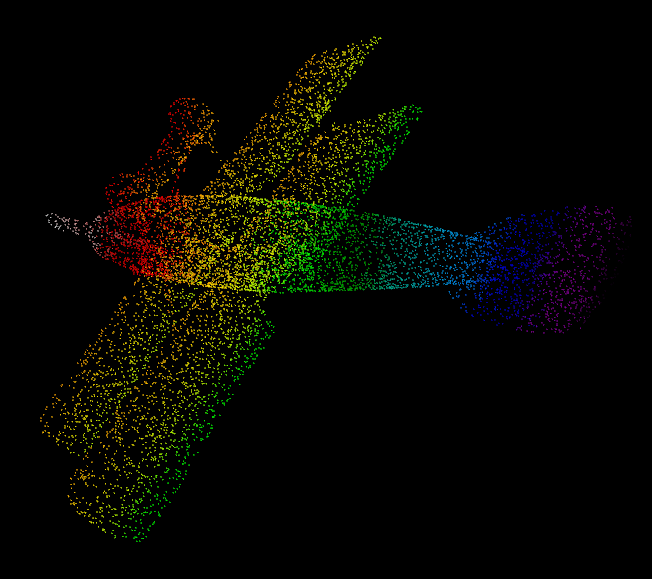# 方案二、matplotlib可视化点云

代码如下所示：

import matplotlib.pyplot as plt
import numpy as np

def viz_matplot(points):
x = points[:, 0]  # x position of point
y = points[:, 1]  # y position of point
z = points[:, 2]  # z position of point
fig = plt.figure()
ax.scatter(x,   # x
y,   # y
z,   # z
c=z, # height data for color
cmap='rainbow',
marker="x")
ax.axis()
plt.show()

viz_matplot(points)


显示结果：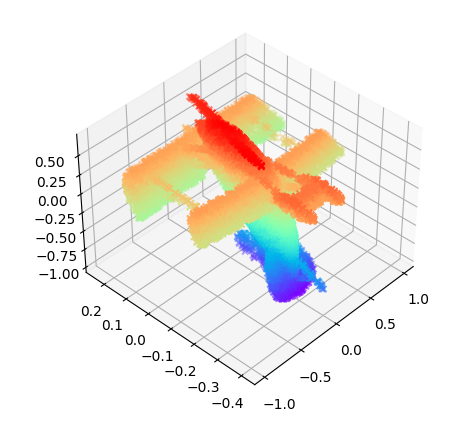# 方案三、CloudCompare可视化点云

直接用软件打开，无需安装。CloudCompare下载地址：CloudCompare点云可视化软件-深度学习文档类资源-CSDN下载

显示结果：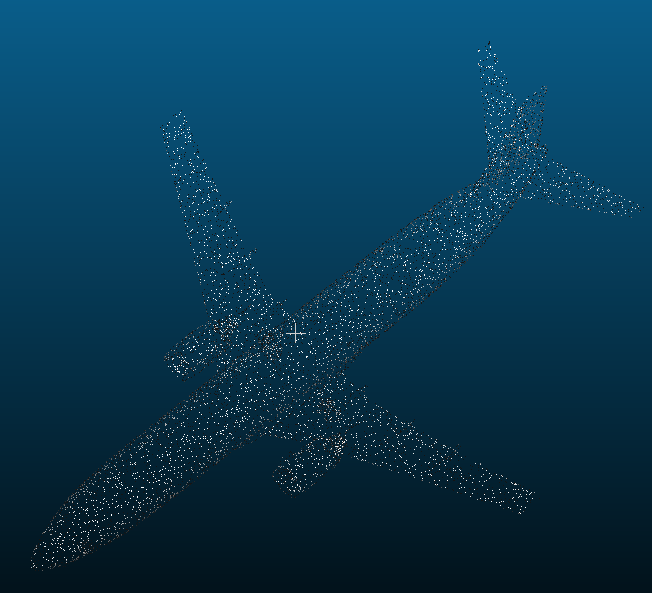# Mayavi常用函数

from mayavi import mlab

fig = mlab.figure(bgcolor=(0, 0, 0), size=(640, 360)) #指定图片背景和尺寸

mlab.points3d(x, y, z,
z,          # Values used for Color，指定颜色变化依据
mode="point",
colormap='spectral', # 'bone', 'copper', 'gnuplot'
# color=(0, 1, 0),   # 也可以使用固定的RGB值
)

opacity=1.0  # 不透明度，取值范围0-1。0.0表示完全透明，1.0表示完全不透明
color=(1, 1, 1)  # RGB数值，每个数的取值范围均为0-1。例：(1, 1, 1)表示白色。
colormap='spectral'  #  不同的配色方案

mlab.show()#显示结果

# 2D data
img = xxxx  # img is a 2D nunmpy array
mlab.imshow(img) #显示二维结果
mlab.surf()
mlab.contour_surf()
mlab.mesh()             #将物体表面以网格（mesh）的形式展示出来，即坐标空间的网格化。# 参数:representation = 'wireframe' 可以仅绘制线框。
# 参数:representation = 'surface' 为default值，绘制完整曲面。mlab.mesh(x, y, z, representation='wireframe', line_width=1.0 )展开全文点云 mayavi...